# How to use the SUMPRODUCT Excel functionYou might think the SUMPRODUCT Excel function is pretty boring at first glance, but the function is actually extremely useful and versatile. While its main purpose is to multiply and add up data in arrays, you can also use it for a wide range of more complicated applications. With that being said, I’ll dive right into the details of this function.

### Syntax

=SUMPRODUCT(array1, [array2], …)
• array1 is the first array that you want to multiply with the other arrays and then add the results
• array2 and all other arrays onward are optional inputs. The function will multiply the components of all the arrays and then sum up the results

### How to use SUMPRODUCT in Excel

I’ll start off by showing a basic example of the SUMPRODUCT Excel function. Like I mentioned before, the function is primarily used to multiply arrays and sum the results.

Take a look at the example below. I have some sales data from a fruit stand.

To find the total revenue for the day, I need to multiply the price and the quantity for each item. Once I do that, I can add up the results, which give me the total revenue.

I did this in column F, but you can see that this method requires a new column and a bunch of individual calculations. The SUMPRODUCT function is a much quicker way to solve this!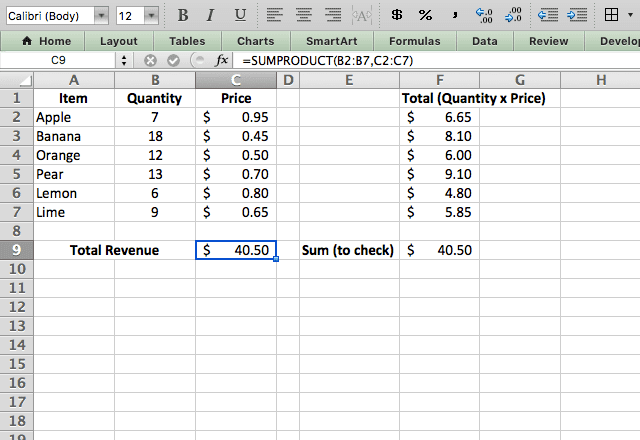By typing =SUMPRODUCT(B2:B7,C2:C7) I instruct the function to multiply each quantity with the corresponding price, and add up the total. This gives me the revenue all in one cell!

I added the longer calculations in column F so you can see that the result is the same – SUMPRODUCT is just much faster.

#### Using SUMPRODUCT to count

When you input multiple arrays, SUMPRODUCT will multiply and sum them. But what if you only input one array? As you saw in the syntax, only the first array is required for the function to work. When you only input one array, the function will simply add up that array. You can see that in the screenshot below, where I use the function =SUMPRODUCT(B2:B7) to add up the total quantity of items sold.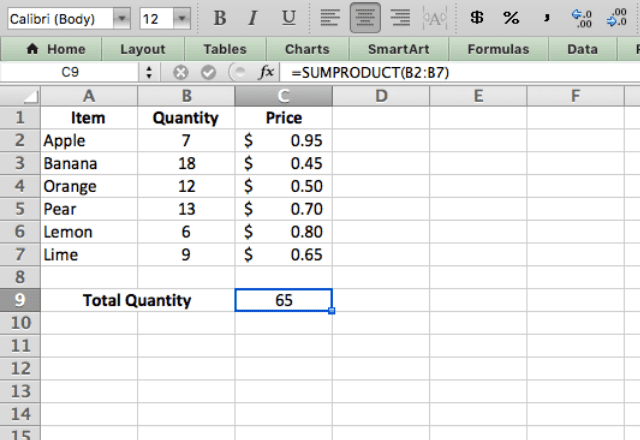In this case, SUMPRODUCT works just like the SUM function!

#### Combining with other functions

One of the benefits of the SUMPRODUCT function is that you can combine it with other functions.

In the example below, I combine it with the LEN function to find the total number of characters with the formula =SUMPRODUCT(LEN(A2:A7)).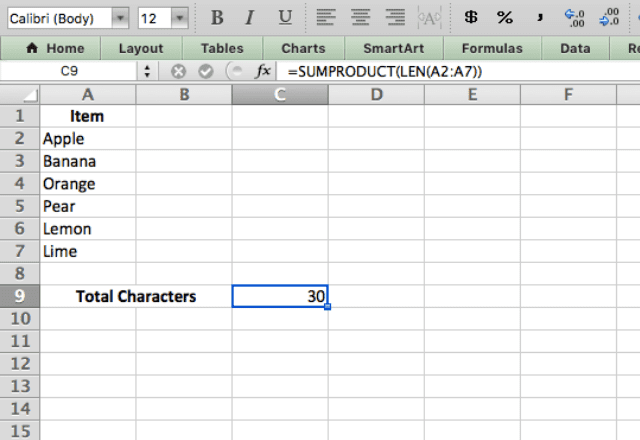#### Other operators

Based on its name, you may think the SUMPRODUCT function can only do multiplication between arrays. However, the function can also do arithmetic operations (*, /, +, -) between arrays. The constant theme is that it always performs the operation across the arrays, and then adds up the total. Take a look at the example below. I use the SUMPRODUCT function to add up the Price and Tax columns, then multiply by the quantity. I do this with the formula =SUMPRODUCT(B2:B7*(C2:C7+D2:D7))./p>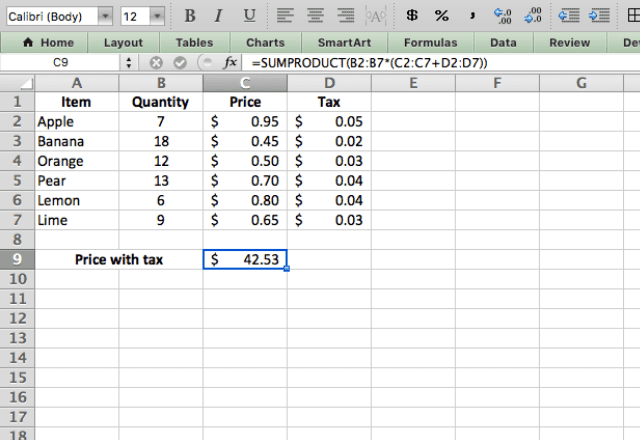Tip: to specify which operation you want to perform, just add the sign between the arrays instead of using a comma. It’s also a good idea to use brackets to make sure it does the operations in the desired order.

#### Count with text

I’ve used SUMPRODUCT with arrays of numbers, but now I’ll demonstrate how you can use it with text.

In this example, I use the Item column as my array, but I also specify that I only want cells with the text string “Banana” (I added another row of bananas just for this example).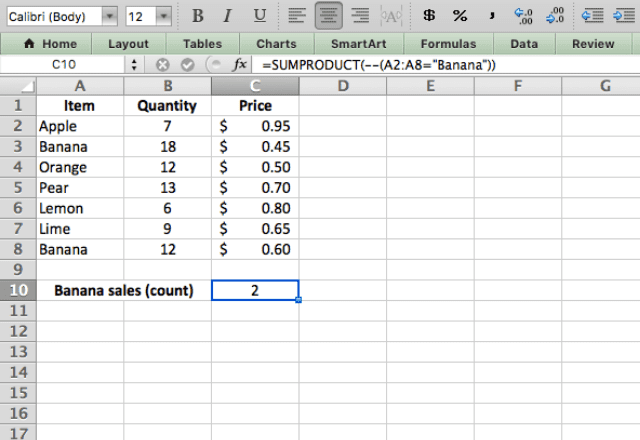You’ll notice something weird about the formula used here, =SUMPRODUCT(–(A2:A8=”Banana”)). It has two negative signs (–) before the brackets. Don’t get too hung up on this – it’s just what the formula needs to compute the results I want.

When SUMPRODUCT evaluates the expression A2:A8=”Banana” it goes down the column and assigns a TRUE or FALSE to each cell. To sum these, I need to convert them from TRUE/FALSE to a number.

The “–“ is like multiplying by -1 twice: once to convert the TRUE/FALSE into a number, and another time to cancel out the negative. So TRUE becomes 1, and FALSE becomes 0. The function then adds these up, and correctly indicates that there are 2 Banana entries.

Note that you could achieve the same effect by using 1* instead of — (i.e. multiply by 1 instead of by -1 twice), but it’s a bit quicker to type the two dashes.

#### SUMPRODUCT with text string and multiple arrays

Now I’ll combine some of the previous examples. Here, I want the total revenue from selling bananas. I have to use 3 different arrays here.

The first looks at the text strings in the Item column and tells the function to only consider bananas (by assigning them a 1 and the rest a 0). Then it multiplies this by the price and quantity, and adds up the result to give the total revenue from bananas.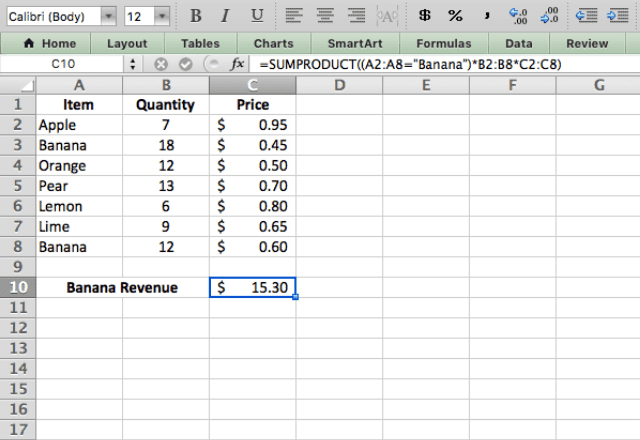The formula here is =SUMPRODUCT((A2:A8=”Banana”)*B2:B8*C2:C8). Note that unlike the last example, I don’t need a — before the first array. That’s because the TRUE/FALSE generated by A2:A8=”Banana” is converted to a number when multiplied with the other arrays. So I only needed that trick when it was for just one array! This example really demonstrates the power of the SUMPRODUCT function. It performs the operations that I want on each entry in my data, and also selectively filters this to give only the results from the items I want to include. All from one formula! I hope that you now see the utility of the SUMPRODUCT function, and how to use it to perform complex calculations with one simple formula!